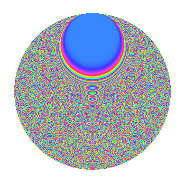# Properties

 Label 2304.2.btLevel $2304$ Weight $2$ Character orbit 2304.bt Rep. character $\chi_{2304}(37,\cdot)$ Character field $\Q(\zeta_{64})$ Dimension $5088$ Sturm bound $768$

# Related objects

## Defining parameters

 Level: $$N$$ $$=$$ $$2304 = 2^{8} \cdot 3^{2}$$ Weight: $$k$$ $$=$$ $$2$$ Character orbit: $$[\chi]$$ $$=$$ 2304.bt (of order $$64$$ and degree $$32$$) Character conductor: $$\operatorname{cond}(\chi)$$ $$=$$ $$256$$ Character field: $$\Q(\zeta_{64})$$ Sturm bound: $$768$$

## Dimensions

The following table gives the dimensions of various subspaces of $$M_{2}(2304, [\chi])$$.

Total New Old
Modular forms 12416 5152 7264
Cusp forms 12160 5088 7072
Eisenstein series 256 64 192

## Trace form

 $$5088q + 32q^{2} - 32q^{4} + 32q^{5} - 32q^{7} + 32q^{8} + O(q^{10})$$ $$5088q + 32q^{2} - 32q^{4} + 32q^{5} - 32q^{7} + 32q^{8} - 32q^{10} + 32q^{11} - 32q^{13} + 32q^{14} - 32q^{16} + 32q^{17} - 32q^{19} + 32q^{20} - 32q^{22} + 32q^{23} - 32q^{25} + 32q^{26} - 32q^{28} + 32q^{29} - 32q^{31} + 32q^{32} - 32q^{34} + 32q^{35} - 32q^{37} + 32q^{38} - 32q^{40} + 32q^{41} - 32q^{43} + 32q^{44} - 32q^{46} + 32q^{47} - 32q^{49} + 32q^{50} - 32q^{52} + 32q^{53} - 32q^{55} + 32q^{56} - 32q^{58} + 32q^{59} - 32q^{61} + 32q^{62} - 32q^{64} + 32q^{65} - 32q^{67} + 32q^{68} - 32q^{70} + 32q^{71} - 32q^{73} + 32q^{74} - 32q^{76} + 32q^{77} - 32q^{79} + 32q^{80} - 32q^{82} + 32q^{83} - 32q^{85} + 32q^{86} - 32q^{88} + 32q^{89} - 32q^{91} + 32q^{92} - 32q^{94} + 32q^{95} - 32q^{97} + 32q^{98} + O(q^{100})$$

## Decomposition of $$S_{2}^{\mathrm{new}}(2304, [\chi])$$ into newform subspaces

The newforms in this space have not yet been added to the LMFDB.

## Decomposition of $$S_{2}^{\mathrm{old}}(2304, [\chi])$$ into lower level spaces

$$S_{2}^{\mathrm{old}}(2304, [\chi]) \cong$$ $$S_{2}^{\mathrm{new}}(256, [\chi])$$$$^{\oplus 3}$$$$\oplus$$$$S_{2}^{\mathrm{new}}(768, [\chi])$$$$^{\oplus 2}$$

## Hecke characteristic polynomials

There are no characteristic polynomials of Hecke operators in the database